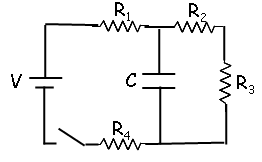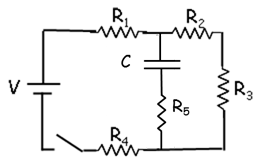# Problem: A circuit is constructed with four resistors, one capacitor, one battery and a switch as shown. The values for the resistors are: R1 = R2 = 45 Ω, R3 = 74 Ω and R4 = 66 Ω. The capacitance is C = 65 uF and the battery voltage is V = 24 V.1. What is Q(∞), the charge on the capacitor after the switch has been closed for a very long time?2. What is I1(0), the magnitude of the current through the resistor R1 just after the switch is closed?3. Continuing with the new circuit, what is Q(∞), the charge on the capacitor after the switch has been closed for a very long time?4. Consider the circuit above, with R5 = 56 Ω in series with the capacitor. Once again, the switch has been open for a long time when at time t = 0, the switch is closed. What is l1(0), the magnitude of the current through the resistor R1 just after the switch is closed?

###### FREE Expert Solution

Charge:

$\overline{){\mathbf{Q}}{\mathbf{=}}{\mathbf{C}}{{\mathbf{V}}}_{{\mathbf{C}}}}$

90% (390 ratings)###### Problem Details

A circuit is constructed with four resistors, one capacitor, one battery and a switch as shown. The values for the resistors are: R1 = R2 = 45 Ω, R3 = 74 Ω and R4 = 66 Ω. The capacitance is C = 65 uF and the battery voltage is V = 24 V.1. What is Q(∞), the charge on the capacitor after the switch has been closed for a very long time?

2. What is I1(0), the magnitude of the current through the resistor R1 just after the switch is closed?

3. Continuing with the new circuit, what is Q(∞), the charge on the capacitor after the switch has been closed for a very long time?

4. Consider the circuit above, with R5 = 56 Ω in series with the capacitor. Once again, the switch has been open for a long time when at time t = 0, the switch is closed. What is l1(0), the magnitude of the current through the resistor R1 just after the switch is closed?